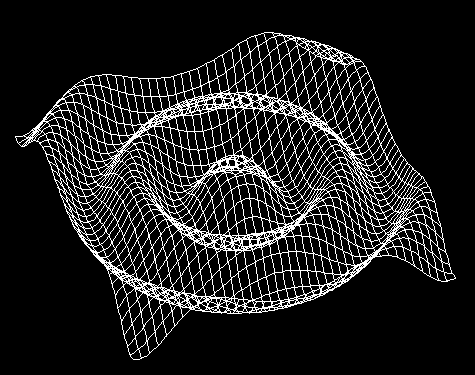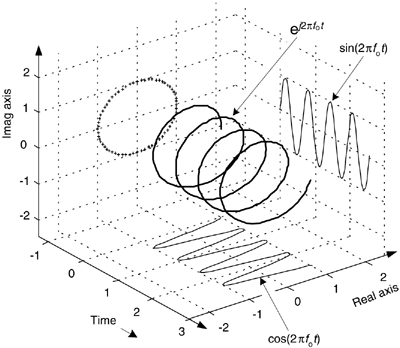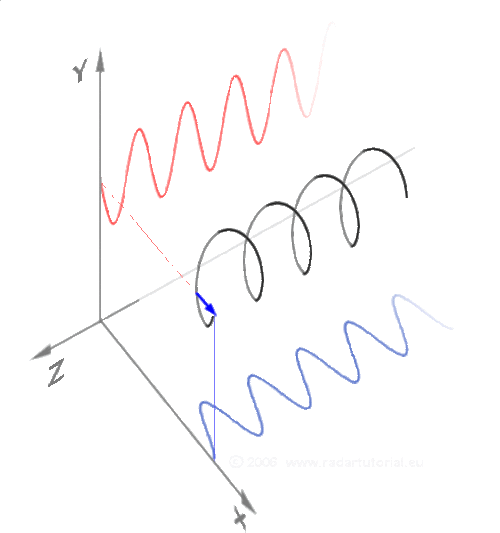# Sine wave

Can someone please give me some code for a 3d sine animation on my 8x8x8 led cube

I already have the led cube's basic output function.

declaring setvoxel(x,y,z); will set a pixel on the cube on.

declaring clrvoxel(x,y,z); will set a pixel on the cube off.

I just need a 3D sine wave code!

sin() and cos(), and oh, I forgot DEG_TO_RAD. That's all you really need to get started.

can you give me the actual code?

circuitdh:
can you give me the actual code?

The thing is, we are here to help, not do the work for you. If you have any trouble or questions, we will help you.
Also, what does this 3d sine wave look like?Or...If you want it to look like the last image, take a closer look at it, it has some useful equations for you. Take a look at the walls of the graph.

Thank you for the help!

So all i have to do is plug the equations into my code like this:

``````for(int x = 0; x < 7; x++){
for(int y = 0; y < 7; y++){
Z = sin(phase + sqrt(pow(map(x,0,7,-PI,PI),2) + pow(map(y,0,7,-PI,PI),2)));
Z = round(map(Z,-1,1,0,7));
setvoxel(x,y,Z);
}
}
``````

My only question now is how would i vary the phase?

thanks for the help!To animate, work out the previous z cooridinate for the x and y and the new coordintate. If they are different, then clear the old one ans set the new one.

If you want it faster, save the coordinates in an array.

Now what I'd like to see is electron orbitals.

PaulMurrayCbr:
To animate, work out the previous z cooridinate for the x and y and the new coordintate. If they are different, then clear the old one ans set the new one.

If you want it faster, save the coordinates in an array.

sorry i don't fully understand what you mean. :{

I haven't taken trig yet...

Maybe this will help:
x: sin wave
y: cos wave
z: time

If you think about it, if you just consider 1 dimension then you will get a sin wave, if you consider 2 dimensions then you get a circle, and if you consider 3 dimensions you get a moving circular spiral.OK so i got that now all i have to do is make it move by varying the phase. A lot of people have told me to vary it between 0 and 2PI, how would i do that? Would i use a for loop and what would i increment it by?

Thanks for all the help and responses! :]

x: sin(time)
y: cos(time)
z: some function that describes distance per time, for example, 1 circle takes 2PI time, so do (2PI * 8 LEDs) / time

This way it will cycle through the circular motion across the cube every 1 second, slow it down by making (2PI * 8) bigger

Ps991:
and if you consider 3 dimensions you get a moving circular spiral.

helix, not spiral. spirals are 2D

sorry but can i please have a small example code? How would i increment between 0 and 2PI.
Thanks!

circuitdh:
How would i increment between 0 and 2PI.

``````...
rads += 0.01; //0.01 is just an example, rads is a float
rads -= TWO_PI; //bring it back into the correct domain
...
``````

Here is a 2D example of what i'm tring to accomplish I would like to be able to vary the wave with the slider of a. The output should be between the black lines

Is there an easy way to do this?
Note: Thank you Ps991 for helping me on this project!here is a link for the wave

circuitdh:
OK so i got that now all i have to do is make it move by varying the phase. A lot of people have told me to vary it between 0 and 2PI, how would i do that? Would i use a for loop and what would i increment it by?

Thanks for all the help and responses! :]

``````for (phase = 0; phase<TWO_PI ; phase+=increment) { /...
``````

The amount of increment really depends on how rapidly you want it to change.

So, I got bored and decided that the best way to show you was to build my own led cube and program it for you, but I didn't want it to be a wussy cube, so I made it RGB to make it pop. Anyways checks to see if anybody believed that story

``````void sineWave3d(){
byte y;
float t = 0;
while(1){
for(int x = 0; x < 8; x++){
for(int z = 0; z < 8; z++){
rads = ((float)sqrt((x-4)*(x-4) + (z-4)*(z-4)) / 4.) * 3 + t; //change that 3 to make it shrink/expand
t += 0.005; //time, change this to make it speed up/slow down
while(t > TWO_PI) //limit to 2PI, wrap around if overflow
t -= TWO_PI;
while(rads > TWO_PI) //limit to 2PI, wrap around if overflow
setLED(x, y[x][z] , z, 0, 0, 0); //clear old LED
y[x][z] = 4 + sin(rads) * 3.999; //this is where the magic happens
setLED(x, y[x][z], z, r, g, b);
}
}
delay(50);
}
}
``````

This works because the LED chosen to light up is based on the distance from the center, that distance is put into the sine function. Then a time variable is added, which causes an animation. If you want to make more sense of it, convert this code for yourself and simply comment "t += 0.005" which will make it all stand still.THANK YOU SO MUCH! IT WORKED!

my last and final question is how would i alter the direction/axis of the sine wave? (its going side to side instead of up/down)

THAN YOU so much for the helpcircuitdh:
THANK YOU SO MUCH! IT WORKED!

my last and final question is how would i alter the direction/axis of the sine wave? (its going side to side instead of up/down)

THAN YOU so much for the helpPost your code, because if it is going side to side, you are doing something wrong.

``````setLED(x, y[x][z], z, r, g, b);
``````

This code right here should only variate the y position, which makes me believe that your setVoxel function is mixing up the y position with the x or z position...

Here is my Code

The set voxel and clr voxel functions work fine!

Thanks for the helpPermission needed, access requested

may I also bring your attention to pastebin.com